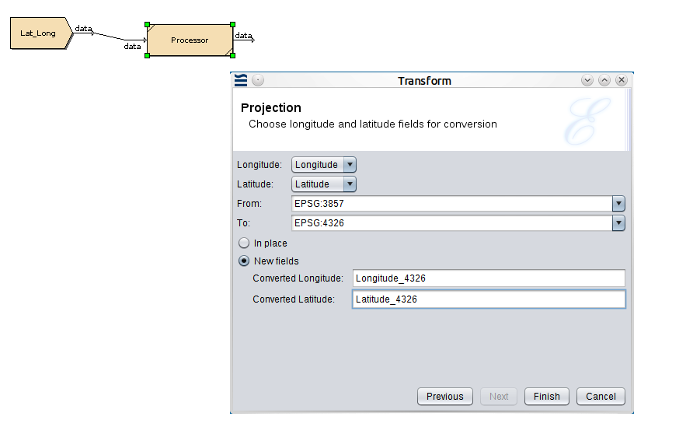## Chapter 5. Projection Transform

Latitude and Longitude Conversion

## Latitude and Longitude Conversion

The Projection Transform converts latitude and longitude values between various geographic coordinate systems.

The Projection Transform currently supports the EPSG: 3857 (http://spatialreference.org/ref/sr-org/6864/) representing Google maps and the EPSG: 4326 (http://spatialreference.org/ref/epsg/wgs-84/) representing Satellite Navigation, coordinate systems.

When working with longitude and latitude, you must know which coordinate systems are being used in your location - for example Ghana uses ESPG:2136, and Hong Kong uses ESRI:104104, but only since 1980. Singapore's official map system is EPSG:3414.

There are two reasons for these different systems - first, the world is not a perfect sphere, so different systems cover different parts of the world where you can approximate to a sphere within a smaller geographical area. Second, projections try to turn 3D information into 2D and this is an approximation - for example, Mercator works well at the equator, but close to the poles is very inaccurate (hence Antarctica looks much bigger than it is).

The latitude and longitude values to convert, must be in numeric format.

The following example shows the Projection Transform and the converted results.

Figure 5.1. Projection TransformFigure 5.2. Projection Transform Results